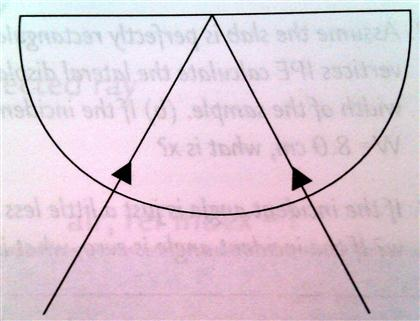# Problem: Given a block of glass that is semi-circular, a laser pointer, protractor/ruler, and index card, design your own experiment to observe and measure total internal reflection. Describe how you would shine the laser onto the glass block, what angles you would measure and how you would determine the critical angle from your measurements. Draw the block of glass and the beam going through it at the critical angle. Show where total internal reflection occurs. (For the homework assume n = 1.52.) Draw this angle and all other as accurately as you can with a protractor. Label the critical angle and give its value. Remember total internal reflection occurs only when light goes from a denser medium (the sample) into one of lower index (air).

###### FREE Expert Solution

This problem requires us to apply experimental techniques to determine critical angle.

Snell's law:

$\overline{){{\mathbf{\eta }}}_{{\mathbf{1}}}{\mathbf{s}}{\mathbf{i}}{\mathbf{n}}{{\mathbf{\theta }}}_{{\mathbf{1}}}{\mathbf{=}}{{\mathbf{\eta }}}_{{\mathbf{2}}}{\mathbf{s}}{\mathbf{i}}{\mathbf{n}}{{\mathbf{\theta }}}_{{\mathbf{2}}}}$

Critical angle:

$\overline{){{\mathbf{\theta }}}_{{\mathbf{c}}}{\mathbf{=}}{\mathbf{s}}{\mathbf{i}}{{\mathbf{n}}}^{\mathbf{-}\mathbf{1}}{\mathbf{\left(}}\frac{{\mathbf{\eta }}_{\mathbf{2}}}{{\mathbf{\eta }}_{\mathbf{1}}}{\mathbf{\right)}}}$

84% (340 ratings)###### Problem DetailsGiven a block of glass that is semi-circular, a laser pointer, protractor/ruler, and index card, design your own experiment to observe and measure total internal reflection. Describe how you would shine the laser onto the glass block, what angles you would measure and how you would determine the critical angle from your measurements. Draw the block of glass and the beam going through it at the critical angle. Show where total internal reflection occurs. (For the homework assume n = 1.52.) Draw this angle and all other as accurately as you can with a protractor. Label the critical angle and give its value. Remember total internal reflection occurs only when light goes from a denser medium (the sample) into one of lower index (air).